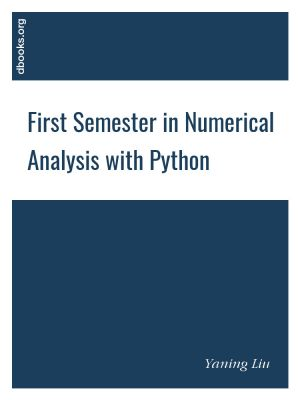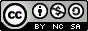# First Semester in Numerical Analysis with Python

by Yaning LiuJoin

### Book Description

The book is based on "First semester in Numerical Analysis with Julia". The contents of the original book are retained, while all the algorithms are implemented in Python (Version 3.8.0). Python is an open source (under OSI), interpreted, general-purpose programming language that has a large number of users around the world. Python is ranked the third in August 2020 by the TIOBE programming community index 2 , a measure of popularity of programming languages, and is the top-ranked interpreted language.

We hope this book will better serve readers who are interested in a first course in Numerical Analysis, but are more familiar with Python for the implementation of the algorithms. The first chapter of the book has a self-contained tutorial for Python, including how to set up the computer environment. Anaconda, the open-source individual edition, is recommended for an easy installation of Python and effortless management of Python packages, and the Jupyter environment, a web-based interactive development environment for Python as well as many other programming languages, was used throughout the book and is recommended to the readers for easy code developement, graph visualization and reproducibility.

Chapter 1
Introduction
Chapter 2
Solutions of equations: Root-finding
Chapter 3
Interpolation
Chapter 4
Chapter 5
Approximation Theory

### Book Details

Title
First Semester in Numerical Analysis with Python
Subject
Computer Science
Publisher
Published
2020
Pages
191
Edition
1
Language
English
PDF Size
6.8 MB### Related BooksThis book presents computer programming as a key method for solving mathematical problems. This second edition of the well-received book has been extensively revised: All code is now written in Python version 3.6 (no longer version 2.7). In addition, the two first chapters of the previous edition have been extended and split up into five new chapte...This book presents computer programming as a key method for solving mathematical problems. There are two versions of the book, one for MATLAB and one for Python. The book was inspired by the Springer book TCSE 6: A Primer on Scientific Programming with Python (by Langtangen), but the style is more accessible and concise, in keeping with the needs o...This book presents computer programming as a key method for solving mathematical problems. There are two versions of the book, one for MATLAB and one for Python. The book was inspired by the Springer book TCSE 6: A Primer on Scientific Programming with Python (by Langtangen), but the style is more accessible and concise, in keeping with the needs o...This book is assembled from lectures given by the author over a period of 10 years at the School of Computing of DePaul University. The lectures cover multiple classes, including Analysis and Design of Algorithms, Scientific Computing, Monte Carlo Simulations, and Parallel Algorithms. These lectures teach the core knowledge required by any scientis...This easy-to-follow textbook/reference presents a concise introduction to mathematical analysis from an algorithmic point of view, with a particular focus on applications of analysis and aspects of mathematical modelling. The text describes the mathematical theory alongside the basic concepts and methods of numerical analysis, enriched by computer ...Invent Your Own Computer Games with Python teaches you how to program in the Python language. Each chapter gives you the complete source code for a new game, and then teaches the programming concepts from the examples. Games include Guess the Number, Hangman, Tic Tac Toe, and Reversi. This book also has an introduction to making games with 2D graph...